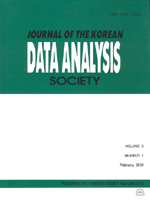상세검색
최근 검색어 전체 삭제
다국어입력
즐겨찾기0KCI등재 학술저널

# Optimal M-level Constant Stress Design with a Polynomial Stress Model for Weibull Distribution

• 등재여부 : KCI등재
• 2004.12
• 1521 - 1529 (9 pages)

Most of the accelerated life tests deal with tests that use only one accelerating variable and no other explanatory variables. Frequently, however, there is a test to use more than one accelerating or other experimental variables, such as, for examples, a test of capacitors at higher than usual conditions of temperature and voltage, a test of circuit boards at higher than usual conditions of temperature, humidity and voltage. In this paper, a accelerated life test is extended to M-level stress accelerated life test with a polynomial stress model. The optimal design for Weibull distribution is studied with a polynomial stress model.

1. Introduction

2. Optimal M-level constant stress design

3. Numerical Examples

References